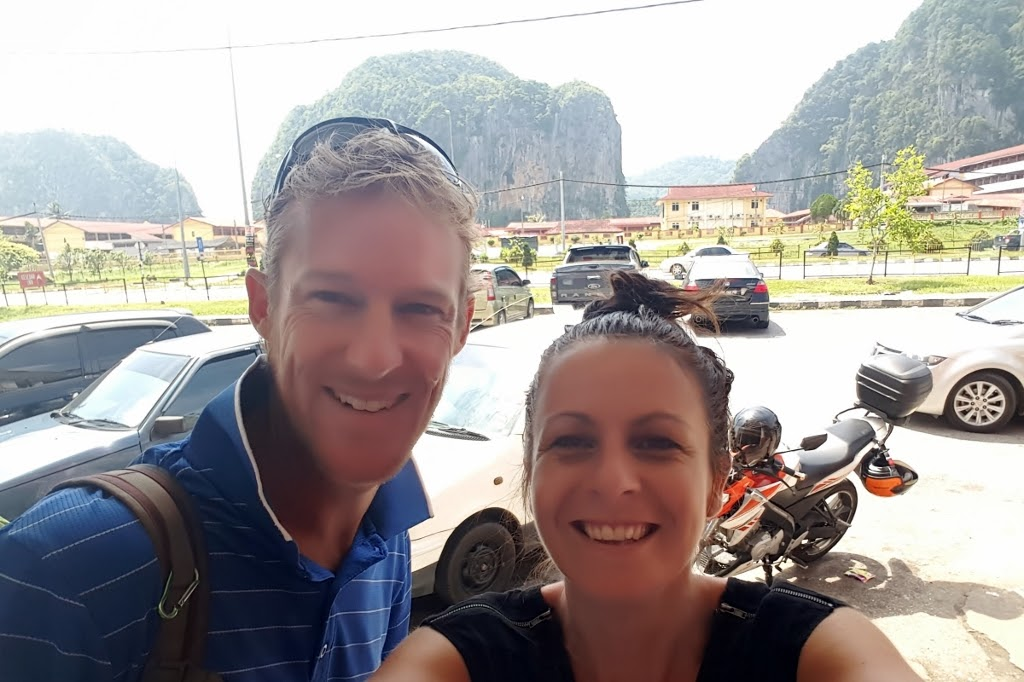# Our DNA: what we do, why and how we do it

Our approach and actions are designed to connect with people not convert or convince.
R
R
R
R
R
R
R
R
R
R
R
R
R
R
R
R
R
R
R
R
R
R
R
R
R
##### We are always learners where listening is as significant and important as sharing